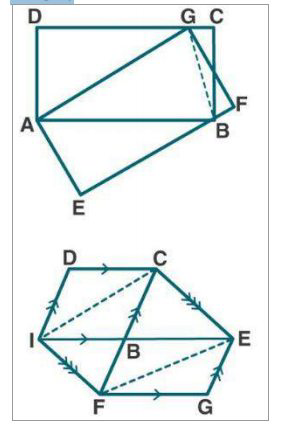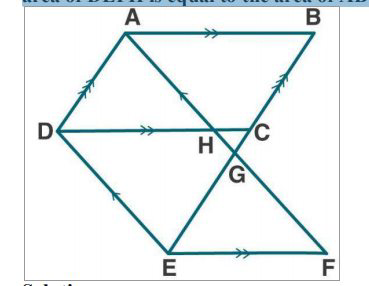# ML Aggarwal Solutions Class 9 Mathematics Solutions for Theorems on Area Exercise 14 in Chapter 14 - Theorems on Area

. (a) In the figure given, ABCD and AEFG are two parallelograms.

Prove that area of || gm ABCD = area of || gm AEFG.

(b) In the fig. (2) Given below, the side AB of the parallelogram ABCD is produced

to E. A straight line through A is drawn parallel to CE to meet CB produced at F

and parallelogram BFGE is Completed prove that area of || gm BFGE=Area of || gm

ABCD.(c) In the figure (3) given below AB || DC || EF, AD || BE and DE || AF. Prove the

area of DEFH is equal to the area of ABCD.(a) Given:

From fig (1)

ABCD and AEFG are two parallelograms as shown in the figure.

To prove:

area of || gm ABCD = area of || gm AEFG

Proof:

let us join BG.

We know that,

ar (∆ABG) = ½ (ar ||gm ABCD) …… (1)

Similarly,

ar (∆ABG) = ½ (ar ||gm AEFG) …. (2)

From (1) and (2)

½ (ar ||gm ABCD) = ½ (ar ||gm AEFG)

So,

ar ||gm ABCD = ar ||gm AEFG)

Hence proved.

(b) Given:

From fig (2)

A parallelogram ABCD in which AB is produced to E. A straight line through A is drawn

parallel to CE to meet CB produced at F and parallelogram BFGE is Completed.

To prove:

area of || gm BFGE=Area of || gm ABCD

Proof:

Let us join AC and EF.

We know that,

ar (∆AFC) = ar (∆AFE) ….. (1)

now subtract ar (∆ABF) on both sides, we get

ar (∆AFC) - ar (∆ABF) = ar (∆AFE) - ar (∆ABF)

Or ar (∆ABC) = ar (∆BEF)

Now multiply by 2 on both sides, we get

1. ar (∆ABC) = 2. ar (∆BEF)

Or ar (||gm ABCD) = ar (||gm BFGE)

Hence proved.

(c) Given:

From fig (3)

AB || DC || EF, AD || BE and DE || AF

To prove:

area of DEFH = area of ABCD

Proof:

We know that,

DE || AF and AD || BE

It is given that ADEG is a parallelogram.

So,

ar (||gm ABCD) = ar (||gm ADEG) ….. (1)

Again, DEFG is a parallelogram.

ar (||gm DEFH) = ar (||gm ADEG) ….. (2)

From (1) and (2)

ar (||gm ABCD) = ar (||gm DEFH)

Or ar ABCD = ar DEFH

Hence proved.

Related Questions
Exercises

Lido

Courses

Teachers

Book a Demo with us

Syllabus

Maths
CBSE
Maths
ICSE
Science
CBSE

Science
ICSE
English
CBSE
English
ICSE
Coding

Terms & Policies

Selina Question Bank

Maths
Physics
Biology

Allied Question Bank

Chemistry
Connect with us on social media!